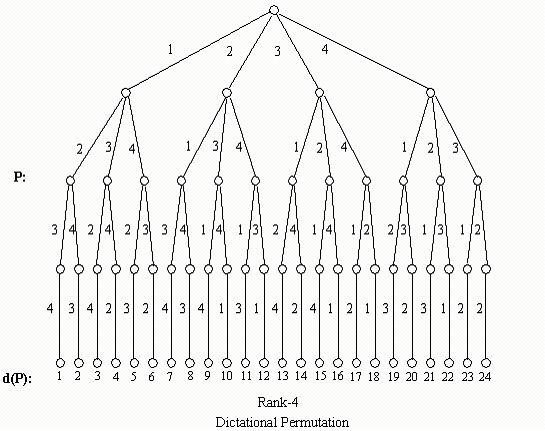Online JudgeProblem SetAuthorsOnline ContestsUser
Web Board
F.A.Qs
Statistical Charts
Problems
Submit Problem
Online Status
Prob.ID:
Register
Authors ranklist
Current Contest
Past Contests
Scheduled Contests
Award Contest
Register
Language:
Coding of Permutations
 Time Limit: 1000MS Memory Limit: 10000K Total Submissions: 1059 Accepted: 440

Description

Write a program that gives us the ordinal position d(P) of any rank-n permutation P=(p1,p2卲n) in the dictionary without producing all the rank-n permutations in order, where pi{1,2,3,...,n},n<=50. When n=4, the whole rank-4 permutation in lexicographical order and the code is shown in the following figure.
For example: if P=(2,3,4,1), then d(P)=10; if P=(4,2,1,3), then d(P)=21.Input

(n, P): For more than one input in the input file, one line is for each input ,with -1 at the end. P is in the form of list.

Output

d(P): It should be in the form of a line with the outputs separated by commas.

Sample Input

```(4,(3,2,1,4))
(5,(3,5,1,2,4))
-1
```

Sample Output

`15,67`

Source

[Submit]   [Go Back]   [Status]   [Discuss]Home PageGo BackTo top